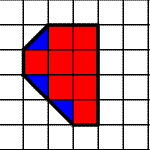# Area

The area of a plane figure, measured in square units (or units 2 ), is the number of squares required to cover it completely .

Example:

The figure shown has an area of $9.5$ square units: $8$ whole squares plus $3$ half-squares.Here are some formulas for the areas of common plane figures.

 Area Formulas Shape Formula Variables Square $A={s}^{2}$ $s$ is the length of the side of the square. Rectangle $A=LW$ $L$ and $W$ are the lengths of the rectangle's sides (length and width). Triangle $A=\frac{1}{2}bh$ $b$ and $h$ are the base and height . Triangle $\begin{array}{l}A=\sqrt{s\left(s-a\right)\left(s-b\right)\left(s-c\right)}\\ \text{Where}\text{\hspace{0.17em}}\text{\hspace{0.17em}}s=\frac{a+b+c}{2}\end{array}$ $a$ , $b$ , and $c$ are the side lengths and $s$ is the semiperimeter Parallelogram $A=bh$ $b$ is the length of the base and $h$ is the height. Trapezoid $A=\frac{{b}_{1}+{b}_{2}}{2}h$ ${b}_{1}$ and ${b}_{2}$ are the lengths of the parallel sides and $h$ the distance (height) between the parallels. Circle $A=\pi {r}^{2}$ $r$ is the radius.